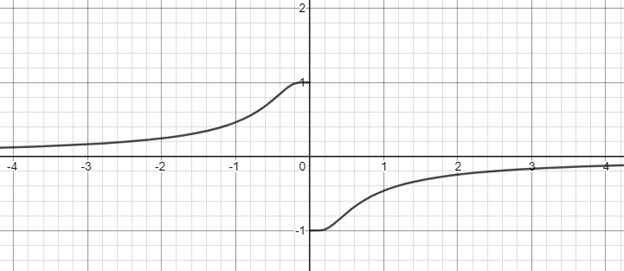# To prove: Thatthe given function is an odd function.### Single Variable Calculus: Concepts...

4th Edition
James Stewart
Publisher: Cengage Learning
ISBN: 9781337687805### Single Variable Calculus: Concepts...

4th Edition
James Stewart
Publisher: Cengage Learning
ISBN: 9781337687805

#### Solutions

Chapter 1.5, Problem 35E
To determine

## To prove: Thatthe given function is an odd function.

Expert Solution

### Explanation of Solution

Given information:

The given function is,

f(x)=1e1/x1+e1/x

Concept used:

Odd function: The graph of the function is symmetric about the origin is called odd function.

Consider the graph of the given function as follows,The graph of the function is symmetric about the origin, so the given function is odd function.

### Have a homework question?

Subscribe to bartleby learn! Ask subject matter experts 30 homework questions each month. Plus, you’ll have access to millions of step-by-step textbook answers!